# Bounds on maximum throughput for digital communications with finite-precision and amplitude constraints

M. Honig, K. Steiglitz, B. Gopinath, and S. Boyd

IEEE Transactions on Information Theory, 36(3):472-484, May 1990.

The problem of finding the maximum achievable data rate over a linear time-invariant channel is considered under constraints different from those typically assumed. The limiting factor is taken to be the accuracy with which the receiver can measure the channel output. More precisely, the following problem is considered. Given a channel with known impulse response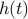, a transmitter with an output amplitude constraint, and a receiver that can distinguish between two signals only if they are separated in amplitude at some time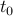by at least some small positive constant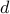, what is the maximum number of messages,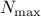, that can be transmitted in a given time interval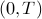? Lower bounds oncan be easily computed by constructing a particular set of inputs to the channel. The main result is an upper bound onfor arbitrary. The upper bound depends on the spread of, which is the maximum range of values the channel output may take at some negative time, given that the output takes on a particular value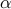at time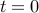. Numerical results are shown for different impulse responses, including two simulated telephone subscriber loop impulse responses.